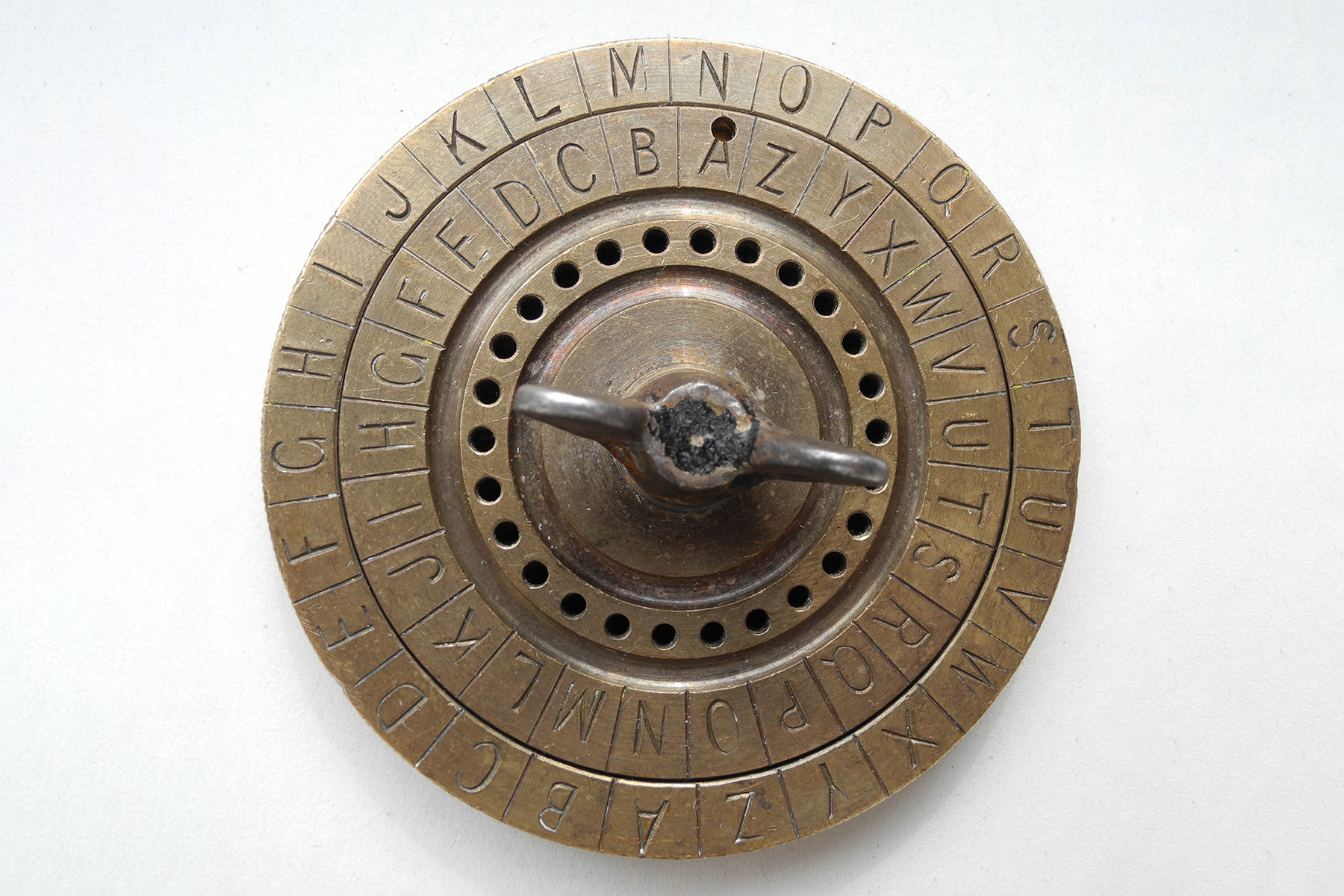## Overview

Caesar cipher is a simple substitution cipher. It rotates the plaintext alphabet by a fixed number of places. The latter is called the “shift” and forms the key of this cipher.

## Example

Assuming the Latin alphabet and a shift of 3 we would replace A with D, B with E, …, Y with B, and Z with C. Try it with the plaintext `ciphereditor`. You should get the ciphertext `jpwolylkpavy`.A construction of two rotating disks with a Caesar cipher can be used to encrypt or decrypt the code (image source)

## How to break the cipher?

The Caesar cipher can be easily broken in a ciphertext-only scenario.

The shift is the key to this cipher and may take up to 25 possible values (assuming the Latin alphabet A-Z with 26 characters). Any other shift value is equivalent to the shift value you get by dividing by the number of characters in the alphabet and taking the remainder (the “modulo operation”). Thus, 260.003 is equivalent to shift 3.

You may break the cipher in a brute-force attack by deciphering the ciphertext at hand using all possible shift values and choosing the original text among them. The latter can be done intuitively or automatically using a dictionary or statistical manners (e.g. frequency analysis).